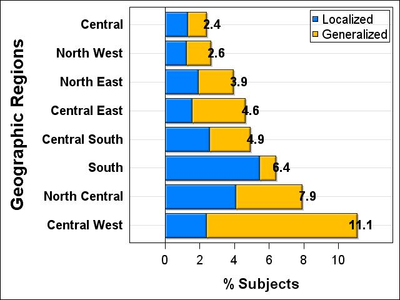## How to align group labels of the hbar in sgplot, and remove space between hbars and labels

Hello,

I am using proc sgplot code below to make this graph. However, I would like the group labels to be left-aligned, and the bars to be close to the group labels (now there is a space). Also, it would be desirable to move the group data values of the hbar a little away from the bars so that they are more readable. I use sas v9.4 (TS1M4).

proc sgplot;

styleattrs datacontrastcolors=(very_dark_blue dark_red)

datacolors=(greenish_Blue "vivid yellowish brown")

datalinepatterns=(solid);

hbarparm category=region3 response=colpercent / group=periodontitis4 grouporder=ascending

scatter x=totpercent y=region3 / markerchar=totpercent

markercharattrs=(size=14 weight=bold) name='scatter';

xaxis discreteorder=data

label='% Subjects'

labelattrs=(size=16pt weight=bold)

valueattrs=(size=14pt);

yaxis grid label='Geographic Regions'

labelattrs=(size=20pt weight=bold)

valueattrs=(size=14pt weight=bold)

display=(noticks) ;

keylegend 'bar' / location=inside position=topright

titleattrs=(weight=bold size=14pt)

valueattrs=(color=black size=14pt)

title=""  down=2;

run;1 ACCEPTED SOLUTION

Accepted SolutionsDanH_sas
SAS Super FREQ

## Re: How to align group labels of the hbar in sgplot, and remove space between hbars and labels

The DATALABEL option did not work because the grouped bar chart is stacked (it would have worked for a "cluster" display). The best way to make this work for you is to use a TEXT plot (see the code below). There are options to adjust the text alignment and for disabling the text plot from contributing to the "min" offset. You want it to contribute to the other offsets so that there is no clipping.

Hope this helps!

Dan

``````proc sgplot;
styleattrs datacontrastcolors=(very_dark_blue dark_red)
datacolors=(greenish_Blue "vivid yellowish brown")
datalinepatterns=(solid);
hbarparm category=region3 response=colpercent / group=periodontitis4 grouporder=ascending
text x=totpercent y=region3 text=totpercent / position=right
contributeoffsets=(xmax ymin ymax)
textattrs=(size=14 weight=bold);
xaxis discreteorder=data
label='% Subjects'
labelattrs=(size=16pt weight=bold)
valueattrs=(size=14pt);
yaxis grid label='Geographic Regions'
labelattrs=(size=20pt weight=bold)
valueattrs=(size=14pt weight=bold)
display=(noticks) ;
keylegend 'bar' / location=inside position=topright
titleattrs=(weight=bold size=14pt)
valueattrs=(color=black size=14pt)
title=""  down=2;
run;``````

8 REPLIES 8Jay54
Meteorite | Level 14

## Re: How to align group labels of the hbar in sgplot, and remove space between hbars and labels

You are using a SCATTER plot overlay to display the totpercent values.  This causes the overlap on the values on the top of the bar.  This is also causing the extra space at the left near the y-axis.  You can use the DATALABEL=totpercent option on the HBARPARM itself and drop the SCATTER statement.

## Re: How to align group labels of the hbar in sgplot, and remove space between hbars and labels

I removed the SCATTER and added the DATALABEL=totpercent.
The extra space is now resolved. However, the total percent is not showing, and the group labels "Central, North West, etc" are not left-aligned. Anything I can do to correct the latter 2 issues?
Thanks.DanH_sas
SAS Super FREQ

## Re: How to align group labels of the hbar in sgplot, and remove space between hbars and labels

The DATALABEL option did not work because the grouped bar chart is stacked (it would have worked for a "cluster" display). The best way to make this work for you is to use a TEXT plot (see the code below). There are options to adjust the text alignment and for disabling the text plot from contributing to the "min" offset. You want it to contribute to the other offsets so that there is no clipping.

Hope this helps!

Dan

``````proc sgplot;
styleattrs datacontrastcolors=(very_dark_blue dark_red)
datacolors=(greenish_Blue "vivid yellowish brown")
datalinepatterns=(solid);
hbarparm category=region3 response=colpercent / group=periodontitis4 grouporder=ascending
text x=totpercent y=region3 text=totpercent / position=right
contributeoffsets=(xmax ymin ymax)
textattrs=(size=14 weight=bold);
xaxis discreteorder=data
label='% Subjects'
labelattrs=(size=16pt weight=bold)
valueattrs=(size=14pt);
yaxis grid label='Geographic Regions'
labelattrs=(size=20pt weight=bold)
valueattrs=(size=14pt weight=bold)
display=(noticks) ;
keylegend 'bar' / location=inside position=topright
titleattrs=(weight=bold size=14pt)
valueattrs=(color=black size=14pt)
title=""  down=2;
run;``````

## Re: How to align group labels of the hbar in sgplot, and remove space between hbars and labels

Dan: Your solution worked nicely. The only change I made is to replace MARKERCHARATTRS with TEXTATTRS because it caused an error.
Thank you very much. The final graph is shown below.DanH_sas
SAS Super FREQ

## Re: How to align group labels of the hbar in sgplot, and remove space between hbars and labels

Sorry about not changing MARKERCHARATTRS to TEXTATTRS. I edited the solution for everyone's future reference. Thanks!

## Re: How to align group labels of the hbar in sgplot, and remove space between hbars and labels

Dan,
One final question: I am placing two versions of this plot side by side, and therefore would like to remove the yaxis labels (valueattrs) "Central, North West, etc" from one of the 2 plots. Is there a way to do that?

JasonDanH_sas
SAS Super FREQ

## Re: How to align group labels of the hbar in sgplot, and remove space between hbars and labels

The DISPLAY option you are using on your YAXIS statement supports up to four options inside the parentheses:

• NOTICKS - remove the tick mark lines
• NOVALUES - remove the tick value text
• NOLINE - remove the axis line
• NOLABEL - remove the axis label

DISPLAY=NONE removes everything. DISPLAY=ALL shows everything. Try these out to get the look you want. As an example, to remove the axis labels and values, you would do DISPLAY=(NOLABEL NOVALUES).

## Re: How to align group labels of the hbar in sgplot, and remove space between hbars and labels

Great. Thank you very much
Discussion stats
• 8 replies
• 1355 views
• 4 likes
• 3 in conversation